# HSSlive: Plus One & Plus Two Notes & Solutions for Kerala State Board

## AP Board Class 10 Physics Chapter 5 Refraction of Light at Plane Surfaces Textbook Solutions PDF: Download Andhra Pradesh Board STD 10th Physics Chapter 5 Refraction of Light at Plane Surfaces Book AnswersAP Board Class 10 Physics Chapter 5 Refraction of Light at Plane Surfaces Textbook Solutions PDF: Download Andhra Pradesh Board STD 10th Physics Chapter 5 Refraction of Light at Plane Surfaces Book Answers

## Andhra Pradesh State Board Class 10th Physics Chapter 5 Refraction of Light at Plane Surfaces Books Solutions

 Board AP Board Materials Textbook Solutions/Guide Format DOC/PDF Class 10th Subject Maths Chapters Physics Chapter 5 Refraction of Light at Plane Surfaces Provider Hsslive

## How to download Andhra Pradesh Board Class 10th Physics Chapter 5 Refraction of Light at Plane Surfaces Textbook Solutions Answers PDF Online?

2. Click on the Andhra Pradesh Board Class 10th Physics Chapter 5 Refraction of Light at Plane Surfaces Answers.
3. Look for your Andhra Pradesh Board STD 10th Physics Chapter 5 Refraction of Light at Plane Surfaces Textbooks PDF.
4. Now download or read the Andhra Pradesh Board Class 10th Physics Chapter 5 Refraction of Light at Plane Surfaces Textbook Solutions for PDF Free.

## AP Board Class 10th Physics Chapter 5 Refraction of Light at Plane Surfaces Textbooks Solutions with Answer PDF Download

Find below the list of all AP Board Class 10th Physics Chapter 5 Refraction of Light at Plane Surfaces Textbook Solutions for PDF’s for you to download and prepare for the upcoming exams:

### 10th Class Physics 5th Lesson Refraction of Light at Plane Surfaces Textbook Questions and Answers

Question 1.
Why is it difficult to shoot a fish swimming in water? (AS1)
(OR)
If the fish is swimming in water, why it is difficult to shoot?
(OR)
A shooter finds it difficult to shoot a fish swimming in water. Why?
Due to refraction of light, it is difficult to shoot a fish swimming in water.

Reason :
The light rays coming from the fish towards shooter, bend at water-air interface. So, shooter sees only image of the fish, but not actual fish.

Question 2.
The speed of light in a diamond is 1,24,000 km/s. Find the refractive index of diamond if the speed of light in air is 3,00,000 km/s. (AS1)
Speed of light in diamond = 1,24,000 km/s
Speed of light in vacuum = 3,00,000 km/s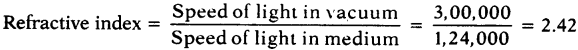Question 3.
Refractive index of glass relative to water is 9/8. What is the refractive index of water relative to glass? (AS1)
Refractive index of glass relative to water = 𝑛𝑔𝑛𝑤=98
∴ Refractive index of water relative to glass = Undefined control sequence \because

Question 4.
The absolute refractive index of water is 4/3. What is the critical angle ? (AS1)
Absolute refractive index of water = 4/3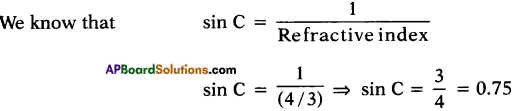Critical angle of water = C = 48°5′ = 48.5°.

Question 5.
Determine the refractive index of benzene if the critical angle is 42°. (AS1)
Critical angle of benzene = 42°.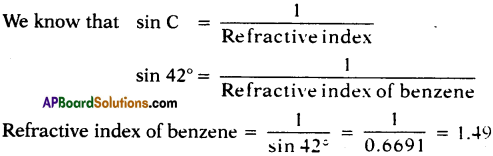Question 6.
Explain the formation of mirage. (AS1)
(OR)
How is the mirage formed? Explain.
(OR)
A person walking on a road observed some water being present on the road but when he went there actually he did not find water. What is that actually formed called? Explain that process.
(OR)
Sometimes during the hot summer at noon time on tar roads, it appears that there is water on the road, but there would really be no water. What do you call this phenomenon? Explain why it happens.
(OR)
Why do you see a mirage the road on a hot summer day?

• During hot summer day, air just above the road surface is very hot and the air at higher altitudes is cool.
• We know that refractive index of air increases with density.
• So, the cooler air at the top has greater refractive index than hotter air just above the road.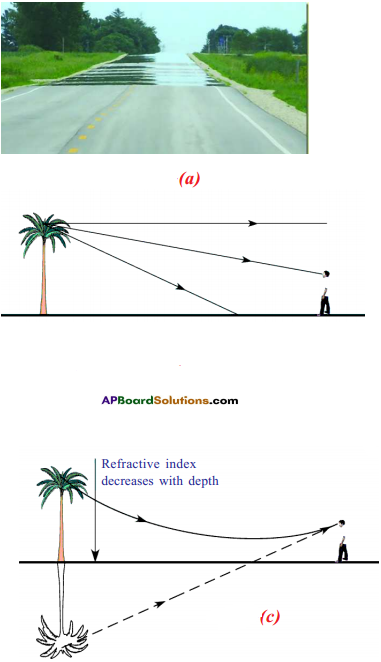• Light travels faster through the thinner hot air than the denser cool air above it.
• On hot days, the temperature decreases with height.
• Thus the refractive index of air increases with height.
• When the light from a tall object such as tree or from the sky passes, through a medium just above the road whose refractive index decreases towards ground, suffers refraction and takes a curved path because of total internal reflection.
• This refracted light reaches the observer in a direction shown as in second figure.
• This appears to the observer that the ray is reflected from ground.
• Hence we will see water on road, which is the virtual image of sky and an inverted image of tree on the road.
• Such virtual images of distant high objects cause the optical illusion called ‘mirage’.

Question 7.
How do you verify experimentally that sin𝑖sin𝑟 is a constant? (AS1)
(OR)
Explain the experiment that shows the relation between angle of incidence and angle of refraction through figure.
(OR)
Write an experiment to obtain the relation between angle of incidence and angle of refraction.
Aim:
Identifying relation between angle of incidence and angle of refraction.

Materials required :
A plank, white chart, protractor, scale, small black painted plank, a semi-circular glass disc of thickness nearly 2 cm pencil and laser light.

Procedure :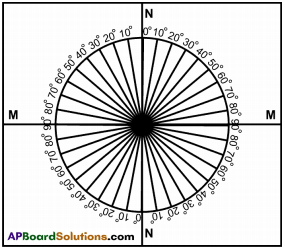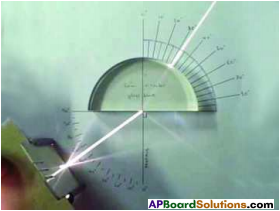1. Take a wooden plank which is covered with white chart.
2. Draw two perpendicular lines, passing through the middle of the paper as shown in the figure (a).
3. Let the intersecting point be O.
4. Mark one line as NN which is normal to the another line marked as MM.
5. Here MM represents the line drawn along the interface of two media and NN represents the normal drawn to this line at ‘O’.
6. Take a protractor and place it along NN in such a way that its centre coincides with ‘O’ as shown in figure (b).
7. Then mark the angles from 0° to 90° on both sides of the line NN.
8. Repeat the same on the other side of the line NN.
9. The angles should be represented on circular line.
10. Now place semi circular glass disc so that its diameter coincides with the interface line (MM) and its centre coincides with the point O.
11. Take the laser light and send it along NN in such a way that the laser propagates from air to glass through the interface at point O and observe the way of laser light coming from other side of disc.
12. There is no deviation.
13. Send Laser light along a line which makes 15° (angle of incidence) with NN and see that it must pass through point O.
14. Measure its corresponding angle of refraction.
15. Repeat the experiment with angle of incidences of 20°, 30°, 40°, 50° and 60° and note the corresponding angles of refraction.

Observation :

• Find sin i, sin r for every i and r note down the values in table.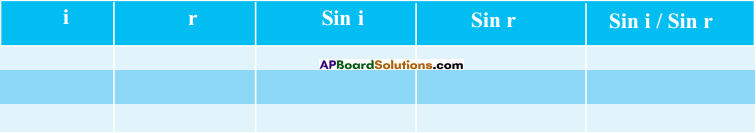• Evaluate sin𝑖sin𝑟 for every incident angle i.
• We get sin𝑖sin𝑟 as constant.
• That is the relationship between angle of incidence and angle of refraction.
• The ratio of sin i and sin r is called refractive index.

Question 8.
Explain the phenomenon of total internal reflection with one or two activities. (AS1)
Procedure :

1. Place the semi-circular glass disc in such a way that its diameter coincides with interlace line MM and its center coincides with point O’.
2. Now send light from the curved side of the semicircular glass disc.
3. The light travels from denser medium to rarer medium.
4. Start with angle of incidence (i), equals to 0° and observe for refracted on other side of the disc.
5. It does not deviate into its path when entering rarer medium.
6. Send laser light along angles of incidence 5°, 10°, 15°, etc. and measure the angle of refraction.
7. And tabulate the results in the given table.

Observation :

• Make a table shown below and note the values ‘i’ and ‘r’.• At particular angle of incidence, the refracted ray does not come out but grazes the interface separating the air and glass. This angle is called critical angle.
• When the angle of incidence is greater than critical angle, the light ray gets reflected into denser medium at the interface, i.e. light never enters rarer medium. This phenomenon is called total internal reflection.

Question 9.
How do you verify experimentally that the angle of refraction is more than angle of incidence when light rays travel from denser to rarer medium? (AS1)
(OR)
When the light rays travel from denser to rarer medium, how can you explain, the angle of refraction is more than angle of incidence experimentally?
Procedure :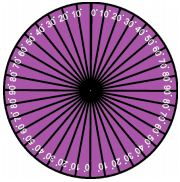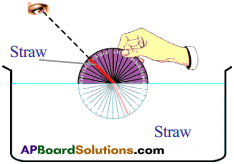• Take a metal disc. Use a protractor and mark angles along its edge as shown in the figure.
• Arrange two straws at the centre of the disc, in such a way that they can be rotated freely about the centre of the disc.
• Adjust one of the straws to make an angle 10°.
• Immerse half of the disc vertically into the water, filled in a transparent vessel. While dipping, verify that the straw at 10° must be inside the water.
• From the top of the vessel, try to view the straw which is inside the water as shown in the figure.
• Then adjust the other straw which is outside of the water until both straws look like they are in a single straight line.
• Then take the disc out of the water and observe the two straws on it. You will find that they are not in a single straight line.
• Measure the angle between the normal and second straw. Note the values in the following table.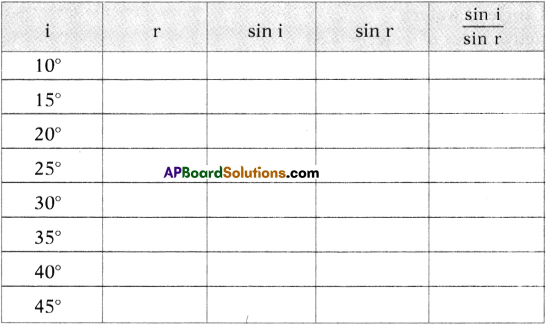• Do the same for various angles and find the corresponding angles of refraction and note them in the table.

Observation :
We will find the angle of refraction is more than angle of incidence.
i. e., r > i.

Conclusion :
When light travels from denser (water) to rarer (air) it bends away from the normal.

Question 10.
Take a bright metal ball and make it black with soot in a candle flame. Immerse it in water. How does it appear and why? (Make hypothesis and do the above experiment) (AS2)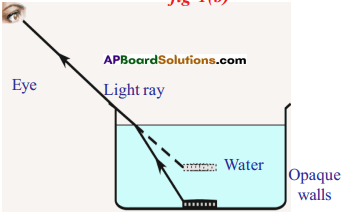• The black metallic .ball appears to be raised up in the water because the path of the ray changes its direction at the interface, separating the two media, i.e., water and air.
• This path is chosen by light ray so as to minimize time of travel between ball and eye.
• This can be possible only when the speed of light changes at interface of two media.
• In another way the speed of light is different in different media.

Hypothesis :
Speed of light changes when it travels from one medium to another medium.

Question 11.
Take a glass vessel and pour some glycerine into it and then pour water up to the brim. Take a quartz glass rod. Keep it in the vessel. Observe the glass rod from the sides of the glass vessel.
1) What changes do you notice?
2) What could be the reasons for these changes? (AS2)

1. We cannot see the glass rod in glycerine but we can see the rod in water.
2. We can also observe an apparent image of glass rod in water.
3. Reasons:
i) Glycerine has essentially same refractive index as glass.
ii) So, any light passing through these is bent equally.
iii) Since both are transparent, it is not possible for our eye to distinguish the boundary by a change in the angle of reflection, and the glass seems to vanish.
iv) But, the refractive index of glass and water are different.
v) So the glass rod is visible to our eye in water. .

Question 12.
Do Activity-7 again. How can you find critical angle of water? Explain your steps briefly. (AS3)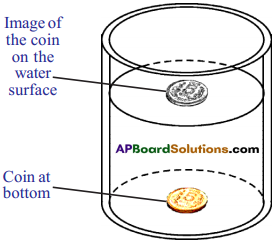Procedure:

1. Take a cylindrical transparent vessel.
2. Place a coin at the bottom of the vessel.
3. Now pour water until you get the image of the coin on the water surface.
4. This is due to total internal reflection.

Critical angle of water :

1. Refractive index of water = 1.33
2. The sine of critical angle of water = 1 Refractive index
3. Sin C = 1 1.33 ⇒ sin C = 0.7518.
∴ C = 8.7°
4. ∴ The critical angle of water = 48.7°.

Question 13.
Collect the values of refractive index of the following media. (AS4)

 Water, coconut oil, flint glass, crown glass, diamond, benzene and hydrogen gas.

 Medium Refractive Index 1. Water 1.33 2. Coconut oil 1.445 3. Flint glass 1.65 4. Crown glass 1.52 5. Diamond 2.42 6. Benzene 1.50 7. Hydrogen gas 1.000132

Question 14.
Collect information on working of optical fibres. Prepare a report about various uses of optical fibres in our daily life. (AS4)
(OR)
What do you know about the working of optical fibres and make a report of various uses of optical fibres in our daily life?
(OR)
How are the optical fibres working? What are the various uses of optical fibres in our daily life?
1) An optical fibre is very thin fibre made of glass or plastic having radius about a micrometer (10-6 m).
2) A bunch of such thin fibres form a light pipe.Working :
1. Optical fibre having three parts-namely core (n = 1, 7), clading (n = 1, 6) and shielding.
2. The ray of light AB gets refracted at point ‘B’ into core and incident at ‘C’ with angle of incidence i (i > c).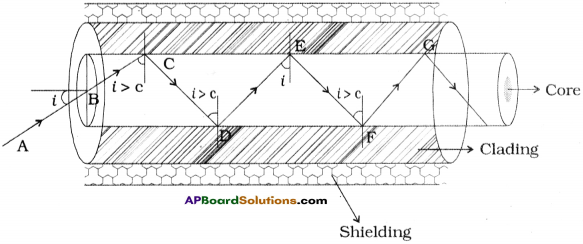3. The angle of incidence is greater than the critical angle and hence total internal reflection takes place.
4. The light is thus transmitted along the fibre.
5. The optical fibre is also based on ‘Fermat’s principle.

Uses :

1. Optical fibres are used in ‘endoscopy’ to see the internal organs like throat, stomach, intestines, etc.
2. Optical fibres are used in transmitting communication signals through light pipes.
3. Optical fibres are used in international telephone cables laid under the sea, in large computer networks, etc.
4. In optical fibre about 2000 telephone signals appropriately mixed with light waves may be simultaneously transmitted through a typical optical fibre.

Question 15.
Take a thin thermocol sheet. Cut it in circular discs of different radii like 2 cm, 3 cm, 4 cm, 4.5 cm, 5 cm etc. and mark centers with sketch pen. Now take needles of length nearly 6 cm. Pin a needle to each disc at its centre vertically. Take water in a large opaque tray and place the disc with 2 cm radius in such a way that the needle is inside the water as shown in figure. Now try to view the free end (head) of the needle from surface of the water.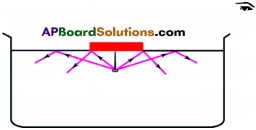1) Are you able to see the head of the needle?
Now do the same with other discs of different radii. Try to see the head of the needle, each time.
Note : The position of your eye and the position of the disc on water surface should not be changed while repeating the Activity with’other discs.
2) At what maximum radius of disc, were you not able to see the free end of the needle ?
3) Why were you not able to view the head of the nail for certain radii of the discs ?
4) Does this Activity help you to find the critical angle of the medium (water) ?
5) Draw a diagram to show the passage of light ray from the head of the nail in different situations. (AS4)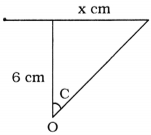1. Yes, we can see head of the needle.

2. Height of the pin = 6 cm
Radius of the disc = x cm
Critical angle of water = C = 48.7°
Tan C = 48.7°
𝑥6 = 1.138 ⇒ x = 6.828 cm
So, at radius of 6.8 cm we cannot see the free end of the needle.

3. Because the light rays coming from object undergoing total internal reflection by touching the surface of disc.

4. Yes, we can find critical angle.
Refractive index of air (n2) = 1.003 ; Refractive index of water (n1) = 1.33
Sin C = 𝑛2𝑛1=1.0031.33 = 0.7541 ⇒ C = 48.7°

5.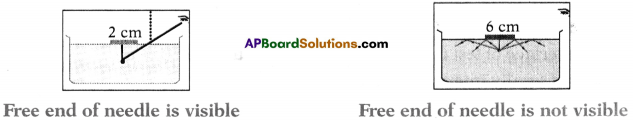Question 16.
Explain the refraction of light through the glass slab with a neat ray diagram. (AS5)
(OR)
Draw a glass slab diagram and explain the refraction of light through glass slab.
(OR)
Write the procedure of a lab Activity to understand lateral shift of light rays through a glass slab.
(OR)
How can you find lateral shift using glass slab?
Aim :
A) Determination of position and nature of image formed by a glass slab.
B) Understanding lateral and vertical shift.
C) Determination of refractive index of given glass slab.

Materials required :
Plank, chart paper, clamps, scale, pencil, thin glass slab and pins.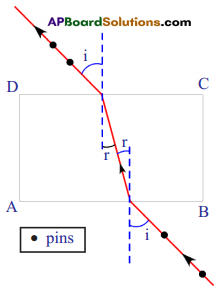Procedure :

1. Place a piece of chart on a plank. Clamp it. Place a glass slab in the middle of the paper.
2. Draw border line along the edges of the slab by using a pencil. Remove it. You will get a figure of a rectangle.
3. Name the vertices of the rectangle as A, B, C and D.
4. Draw a perpendicular at a point on the longer sides (AB) of the rectangle.
5. Now draw a line, from the point of intersection where side AB of rectangle and perpendicular meet, in such a way that it makes 30° angle with the normal.
6. This line represents the incident ray falling on the slab and the angle it makes with normal represents angle of incidence.
7. Now place the slab on the paper in such a way that it fits in the rectangle drawn. Fix two identical pins on the line making 30° angle with normal, such that they stand vertically with same height.
8. By looking at the two pins from the other side of the slab, fix two pins in such a way that all pins appear to be along a straight line.
9. Remove the slab and take out pins. Draw a straight line by joining the dots formed by the pins up to the edge CD of the rectangle.
10. This line represents emergent ray of the light.
11. Draw a perpendicular to the line CD where our last line drawn meets the line CD.
12. Measure the angle between emergent ray and normal.
13. This is called angle of emergence.
14. The angle of incidence and angle of emergence are equal.
15. Incident emergent rays are parallel.
16. The distance between the parallel rays is called shift.

Question 17.
Place an object on the table. Look at the object through the transparent glass slab. You will observe that it will appear closer to you. Draw a ray diagram to show the passage of light ray in this situation. (AS5)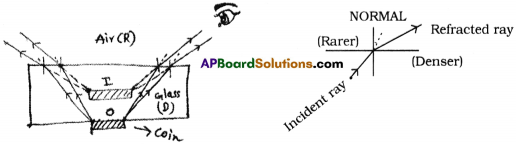Question 18.
What is the reason behind the shining of diamond and how do you appreciate it? (AS6)
(OR)
For which reason is the diamond shining and how is it appreciable?

• The critical angle of diamonds is very low, i.e., 24.4°.
• So if a light ray enters diamond, it undergoes total internal reflection.
• It makes the diamond shine brilliant.
• So total internal reflection is main cause of brilliance of diamonds.
• Majority of people are attracted towards diamonds due to this property.
• So we have to thoroughly appreciate total internal reflection for brilliance of diamonds.

Question 19.
How do you appreciate the role of Fermat’s principle in drawing ray diagrams? (AS6)
(OR)
How do you admire the role of Fermat’s principle in drawing ray diagrams? Fermat’s principle: The light ray always travels in a path which needs shortest possible time to cover distance between two points.
This principle has lot of importance on optics. This is used in

1. Laws of reflection (i.e., angle of incidence = angle of reflection)
2. Laws of refraction (Snell’s law)
3. To derive refractive index of a medium.
4. To derive refractive index of glass slab.
So, I appreciate the Fermat’s principle.

Question 20.
A light ray is incident on air-liquid interface at 45° and is refracted at 30°. What is the refractive index of the liquid? For what angle of incidence will the angle between reflected ray and refracted ray be 90°? (AS7)
i) Given that angle of incidence (i) = 4S°
angle of refraction (r) = 30°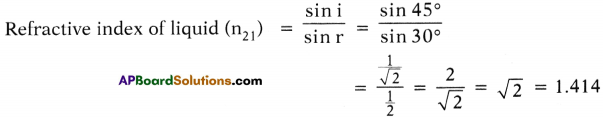ii) Given that angle between reflected and refracted ray is 90°.
We know angle of incidence = angle of reflection
∴ Angle of refraction (r) = 90 – angle of incidence
= 90 – i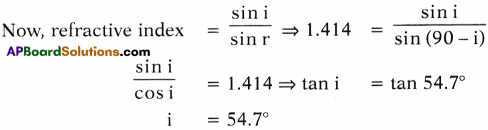Critical angle = 54.7°. This angle is also known as polarising angle.

Question 21.
Explain why a test tube immersed at a certain angle in a tumbler of water appears to have a mirror surface from a certain viewing position. (AS7)
When a test tube is immersed at a certain angle in a tumbler of water appears to have
a mirror surface from a certain viewing positions due to total internal reflection.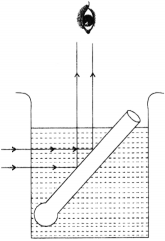Explanation :

• The critical angle for glass is 42°.
• The glass and air in test tube works as denser and rarer mediums.
• The rays of light while travelling through water strike glass – air interface of test tube at an angle of more than 42° (i > c) they get totally internal reflected as shown figure.
• When these reflected rays reach the eye, they appear to come from the surface of test tube itself.
• Now the test tube appears like silvary.

Question 23.
In what cases does a light ray not deviate at the interface of two media? (AS7)

1. When a light ray incident is perpendicular to the interface of surface, it does not undergo deviation.
2. When a light ray incident is more than critical angle, it does not undergo deviation (refraction) but it undergoes reflection to come back into the original medium.

Question 25.
When we sit at camp fire, objects beyond the fire seen swaying. Give the reason for it. (AS7)
(OR)
What are the reasons for the objects beyond the fire seen swaying, when we sit at camp fire?

• The temperature of the surrounding air changes due to convection of heat by the camp fire.
• This leads to chang in density and refractive index of air, continuously.
• The continuous change in refractive index of air changes the refracted path of the light ray.
• This is the cause for swaying of an object.

Question 26.
Why do stars appear twinkling? (AS7)
(OR)
What is the reason for the appearance of stars like twinkling?

• The twinkling of a star is due to atmospheric refraction of star light.
• The atmosphere consists of a number of layers of varying densities.
• When light rays coming from a star pass through this layers and undergo refraction for several times.
• Thats why stars appear twinkling.

Question 27.
Why does a diamond shine more than a glass piece cut to the same shape? (AS7)
(OR)
What is the reason for shining of diamond brightly as compared to glass piece cut?

• The critical angle of a diamond is very low (i.e., 24.4°).
• So if a light ray enters a diamond it definitely undergoes total internal reflection.
• Whereas it is not possible with glass piece cut to the same shape.
• So diamond shines more than a glass piece.

Fill In The Blanks

1. At critical angle of incidence, the angle of refraction is ……………… .
2. n1 sin i = n2 sin r, is called ……………… .
3. Speed of light in vacuum is ……………… .
4. Total internal reflection takes place when a light ray propagates from …………. to …………… medium.
5. The refractive index of a transparent material is 3/2. The speed of the light in that medium is …………… .
6. Mirage is an example of ……………… .

1. 90°
2. Snell’s law
3. 3 × 108 m/s
4. denser, rarer
5. 2 × 108 m/s
6. optical illusion / total internal reflection

Multiple Choice Questions

1. Which of the following is Snell’s law?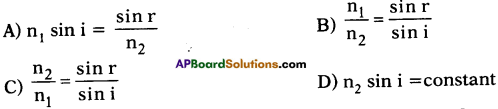B)

2. The refractive index of glass with respect to air is 2. Then the critical angle of glass air interface is ………………….
A) 0°
B) 45°
C) 30°
D) 60°
C) 30°

3. Total internal reflection takes place when the light ray travels from …………….. .
A) rarer to denser medium
B) rarer to rarer medium
C) denser to rarer medium
D) denser to denser medium
C) denser to rarer medium

4. The angle of deviation produced by the glass slab is …………… .
A) 0°
B) 20°
C) 90°
D) depends on the angle formed by the light ray and normal to the slab
D) depends on the angle formed by the light ray and normal to the slab

### 10th Class Physics 5th Lesson Refraction of Light at Plane Surfaces Additional Questions and Answers

Question 1.
Derive Snell’s law.
(OR)
Prove n1 sin i = n2 sin r.
(OR)
Derive the Snell’s formula from Fermat’s principle.
(OR)
Derive the formula in realtion with and of incidence and angle of refraction.
Let X be the path and A be the point above X and B be the point below X.
Now, we have to find the way from A to B.

1. Let us try to calculate how long it would take to go from A to B by the two paths through point D and another through point C.
2. If we draw a perpendicular DE, between two paths at D, we see that the path on line is shortened by the amount EC.
3. On the other hand, in the water, by drawing corresponding perpendicular CF we find that we have to go to the extra distance DF in water. These times must be equal since we assumed there was no change in time between two paths.
4. Let the time taken by the man to travel from E to C and D to F be ∆t and v1 and v2 be the speeds of the running and swimming. From figure we get,
EC = v1 ∆t and DF = v2 ∆t
⇒ ECDF=v1v2 ………….. (1)
5. Let i and r be the angles measured between the path ACB and normal NN, perpendicular to shore line X.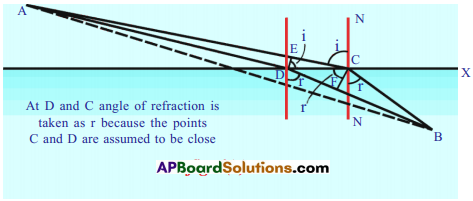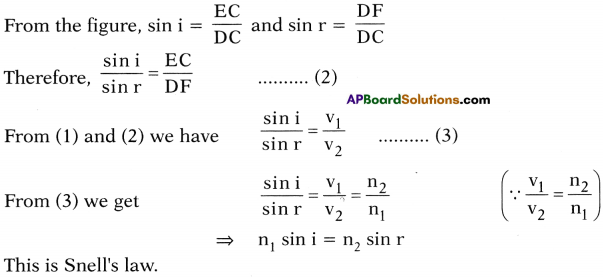### 10th Class Physics 5th Lesson Refraction of Light at Plane Surfaces InText Questions and Answers

10th Class Physics Textbook Page No. 56

Question 1.
Why should you see a mirage as a flowing water?

• A mirage is a naturally occuring optical phenomenon, in which light rays are bent to produce a displaced image of distant objects or the sky.
• As light passes from colder air (higher place) to warmer air (lower place), the light ray bends away from the direction of the temperature gradient.
• Once the rays reach the viewer’s eye, the visual cortex interprets it as if it traces back along a perfectly straight “line of sight”. However this line is at a tangent to the path the ray takes at the point it reaches the eye.
• The result is that an “inferior image ” of the sky above appears on the ground.
• The viewer may incorrectly interprets this sight as water that is reflecting the sky, which is to the brain, a more reasonable and common occurrence.

Question 2.
Can you take a photo of a mirage?

• Yes, I can take a photo of a mirage.
• Our eye can catches the total internal reflected rays.
• So, camera lens also catches the same.

10th Class Physics Textbook Page No. 46

Question 3.
What difference do you notice in fig 2(a) and Fig 2(b) with the respect to refracted rays?
(OR)
Draw the ray diagram of refraction in between denser and rarer medium.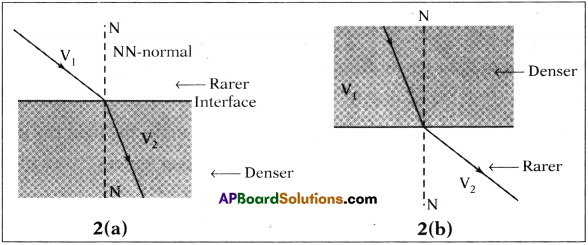In figure 2(a) the light ray bends towards normal whereas in 2(b) the light ray bends away from the normal.

Question 4.
Is there any relation between behaviour of refracted rays and speed of the light?
Yes. The speed of light changes when it travels from one medium to another medium. So the light may bend towards normal or away from normal.

10th Class Physics Textbook Page No. 47

Question 5.
Why db different material media possess different values of refractive indices?
Refractive index depends on nature of material. So different media have different values of refractive indices.

10th Class Physics Textbook Page No. 48

Question 6.
On what factors does the refractive index of a medium depend?
Refractive index depends on (1) Nature of material and (2) Wavelength of light used.

10th Class Physics Textbook Page No. 49

Question 7.
Can we derive the relation between the angle of incidence and the angles of refraction theoretically?
Yes, we can derive the relation between angle of incidence and angles of refraction theoretically. We can get nt sin i = n2 sin r.

10th Class Physics Textbook Page No. 53

Question 8.
Is there any chance that angle of refraction is equal to 90° ? When does this happen?
Yes, when angle of incidence is equal to critical angle then angle of refraction is equal to 90°.

10th Class Physics Textbook Page No. 54

Question 9.
What happens to light when the angle of incidence is greater than critical angle?
When the angle of incidence is greater than critical angle, the light ray gets reflected into denser medium at the interface, i.e., light never enters rarer medium. This phenomenon is called total internal reflection.

10th Class Physics Textbook Page No. 57

Question 10.
How does light behave when a glass slab is introduced in its path?
The light ray undergoes refraction two times.

10th Class Physics 5th Lesson Refraction of Light at Plane Surfaces Activities

Activity – 1

Question 1.
Procedure :
Take some water in a glass tumbler. Keep a pencil in it. See the pencil from one side of glass and also from the top of the glass.
Observation:

1. How does it look?
From the side it appears to be bent. From the top it appears as it is raising.

2. Do you find any difference between two views?
Yes, the position of pencil is different.

Activity – 2

Question 2.
Procedure :

1. Go to a long wall (of length of 30 feet) facing the Sun. Go to the one end of a wall and ask someone to bring a bright metal object near the other end of the wall.
2. When the object is few inches from the wall, it will distort and we will see a reflected image on the wall as though the wall were a mirror.

Observation:

Why is there an image of the object on the wall?
The image is due to refraction of light.

Activity-3 Refraction

Question 3.
Procedure: –

1. Take a shallow vessel with opaque walls such as a mug, a tin or a pan.
2. Place a coin at the bottom of the vessel.
3. Move away from the vessel until we cannot see the coin (fig. 2). Ask someone to fill the vessel with water. When the vessel is filled with water the coin comes back into view (fig. 3).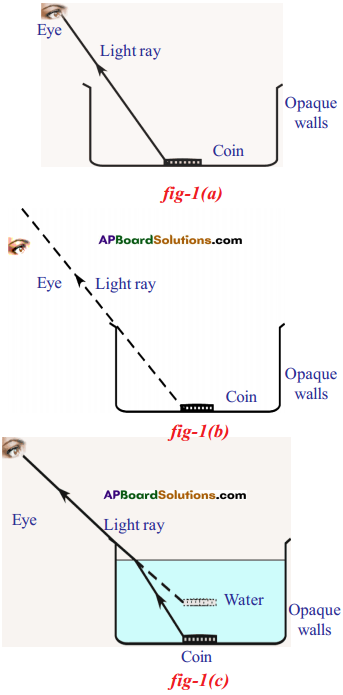1. Why are you able to see the coin when the vessel is filled with water?
The ray of light originated from the coin does not reach your eye when the vessel is empty. Hence you are not able to see the coin. But the coin becomes visible after the vessel is filled with water.

2. How is it possible? Do you think that the ray reaches your eye when the vessel is filled with water?
Yes, it reaches the second instance.

3. What happens to the light ray at interface between water and air?
It bends towards the normal.

4. What could be the reason for this bending of the light ray in the second instance?
It is based on Fermat’s principle, which states that the light ray always travels in a path which needs shortest possible time to cover the distance between the two points.

Activity – 4

Question 4.
Prove that when light ray travels from denser to rarer medium it bends away from the normal.Procedure :

1. Take a metal disc. Use protractor and mark angles along its edge as shown in the figure.
2. Arrange two straws from the centre of the disk.
3. Adjust one of the straws to the angle 10°.
4. Immerse half of the disc vertically into the water, filled in a transparent vessel.
5. Inside the water the angle of straw should be at 10°.
6. From the top of the vessel try to view the straw which is inside the water.
7. Then adjust the other straw which is outside the water until both straws are in a single straight line.
8. Then take the disc out of the water and observe the two straws on it.
9. We will find that they are not in a single straight line.
10. It could be seen from the side view while half of the disc is inside the water.
11. Measure the angle between the normal and second straw. Draw table for various angles and corresponding angles of refraction.

Observation :
We observe that ‘r’ is greater than ‘i’ in all cases and when light travels from
denser to rarer medium it bends away from the normal.

Activity – 6

Question 5.
Why can we not see a coin placed in water from the side of glass?
Procedure :

1. Take a transparent glass tumbler and coin.
2. Place a coin on a table and place glass on the coin.
3. Observe the coin from the side of the glass. We can see the coin.
4. Now fill the glass with water and observe the coin from the side of the glass tumber.
5. Now we cannot see the coin because the coin rises up due to refraction.

Activity – 7

Question 7.
Why can we see the coin in water from top? What is the phenomenon behind that?Procedure :

1. Take a cylindrical transparent vessel. Place a coin at the bottom of the vessel.
2. Now pour water until we will get the image of the coin on the water surface.
3. This is due to total internal reflection.

Activity – 8

Question 8.
Write an Activity to find refractive index of glass slab by calculating vertical shift.
(OR)
Explain the experiment with glass slab in determination of refraction through vertical shift.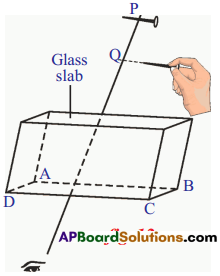Procedure :

1. Take a glass slab and measure the thickness of the slab.
2. Take a white chart and fix it on the table.
3. Place the slab in the middle of the chart.
4. Draw line around it.
5. Remove the slab from its place.
6. The lines form a rectangle. Name the vertices of it as A. B, C and D. ‘
7. Draw a perpendicular to the longer line AB of the rectangle at any point on it.
8. Place slab again in the rectangle ABCD.
9. Take a needle. Place at a point P in such a way that its length is parallel to the AB on the perpendicular line at a distance of 20 cm from the slab.
10. Now take another needle and by seeing at the first needle from the other side of the slab, try to keep the needle so that it forms a straight line with the first needle. 1 1)
11. Remove the slab and observe the positions of the needles.
12. They are not in same line.
13. Draw a perpendicular line from the second needle to the line on which the first needle is placed.
14. Take the intersection point as Q.
15. The distance between P and Q is vertical shift.
16. We will get the same vertical shift placing needle at different distances.
17.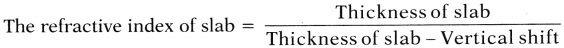## Andhra Pradesh Board Class 10th Physics Chapter 5 Refraction of Light at Plane Surfaces Textbooks for Exam Preparations

Andhra Pradesh Board Class 10th Physics Chapter 5 Refraction of Light at Plane Surfaces Textbook Solutions can be of great help in your Andhra Pradesh Board Class 10th Physics Chapter 5 Refraction of Light at Plane Surfaces exam preparation. The AP Board STD 10th Physics Chapter 5 Refraction of Light at Plane Surfaces Textbooks study material, used with the English medium textbooks, can help you complete the entire Class 10th Physics Chapter 5 Refraction of Light at Plane Surfaces Books State Board syllabus with maximum efficiency.

## FAQs Regarding Andhra Pradesh Board Class 10th Physics Chapter 5 Refraction of Light at Plane Surfaces Textbook Solutions

#### Can we get a Andhra Pradesh State Board Book PDF for all Classes?

Yes you can get Andhra Pradesh Board Text Book PDF for all classes using the links provided in the above article.

## Important Terms

Andhra Pradesh Board Class 10th Physics Chapter 5 Refraction of Light at Plane Surfaces, AP Board Class 10th Physics Chapter 5 Refraction of Light at Plane Surfaces Textbooks, Andhra Pradesh State Board Class 10th Physics Chapter 5 Refraction of Light at Plane Surfaces, Andhra Pradesh State Board Class 10th Physics Chapter 5 Refraction of Light at Plane Surfaces Textbook solutions, AP Board Class 10th Physics Chapter 5 Refraction of Light at Plane Surfaces Textbooks Solutions, Andhra Pradesh Board STD 10th Physics Chapter 5 Refraction of Light at Plane Surfaces, AP Board STD 10th Physics Chapter 5 Refraction of Light at Plane Surfaces Textbooks, Andhra Pradesh State Board STD 10th Physics Chapter 5 Refraction of Light at Plane Surfaces, Andhra Pradesh State Board STD 10th Physics Chapter 5 Refraction of Light at Plane Surfaces Textbook solutions, AP Board STD 10th Physics Chapter 5 Refraction of Light at Plane Surfaces Textbooks Solutions,
Share: